# Quantum Graph Neural Networks Applied

## Tackling particle reconstruction with hybrid quantum-classical graph neural networks

We’ll do an in-depth breakdown of graph neural networks, how the quantum analogue differs, why one would think of applying it to high energy physics, and so much more. This post is for you if:

• if you’re interested in the ins & outs of intriguing QML applications
• you’re keen to get a snapshot understanding of how classical graph neural networks work
• you’re the kind of person that gets dopamine hits when you make cross-domain connections between ideas

Ready to begin? Here’s the map we’ll follow.

1. We’ll start with understanding our problem setting at CERN, touching on what’s being done to solve it right now, and why graphML could be the potential key to solving it.
2. Then we’ll make an interlude to understand classical graph neural networks
3. So that we can break down the quantum graph neural network in detail
4. And end off by sharing how my group & I contributed to the existing work.

Context

When Xanadu’s QHack rolled around in late Feb, my team and I were on the hunt for appealing QML projects. We took one glance at this quantum graph neural network paper and knew it wouldn’t disappoint. Indeed it didn’t.

5 days later, we ended up placing in the top 5 teams and winning several power-ups & prizes along the way.

It was partly because this project was an exotic blend of many things I love.

Graph theory. Machine learning. Quantum Computing.

And it also sparked some new passions: high-energy physics. No less, working on this project taught me a marriage of these concepts. And so in this post, I’d like to share this exotic fusion with you. In detail.

Let me preface that my intention here is not to convince you of the quantum upside — which remains unknown — but rather to journey you through the jungle of learnings reaped from exploring this QML application.

Allons-y!

# Section 1: GraphML meets High Energy Physics

Before diving right into the QML, let’s understand the problem we’re hoping to solve along with the context it resides upon. That takes us to Geneva.

## CERN general operations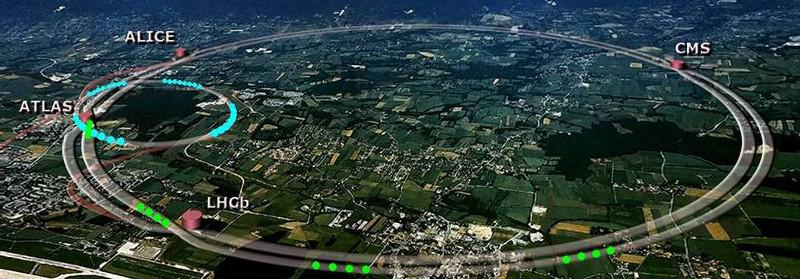Diagram of the particle accelerator rings at CERN (27km in circumference) | Image credit: CERN

The Large Hadron Collider (LHC) in Geneva, Switzerland, is the world’s largest and most powerful particle accelerator. It’s a 27-kilometre ring that uses superconducting magnets along with a number of accelerating structures to boost the energy of the particles along the way.

Given that it took a decade to build and nearly cost around \$4.75B, you may reasonably ask, what does it even do that’s worth so much?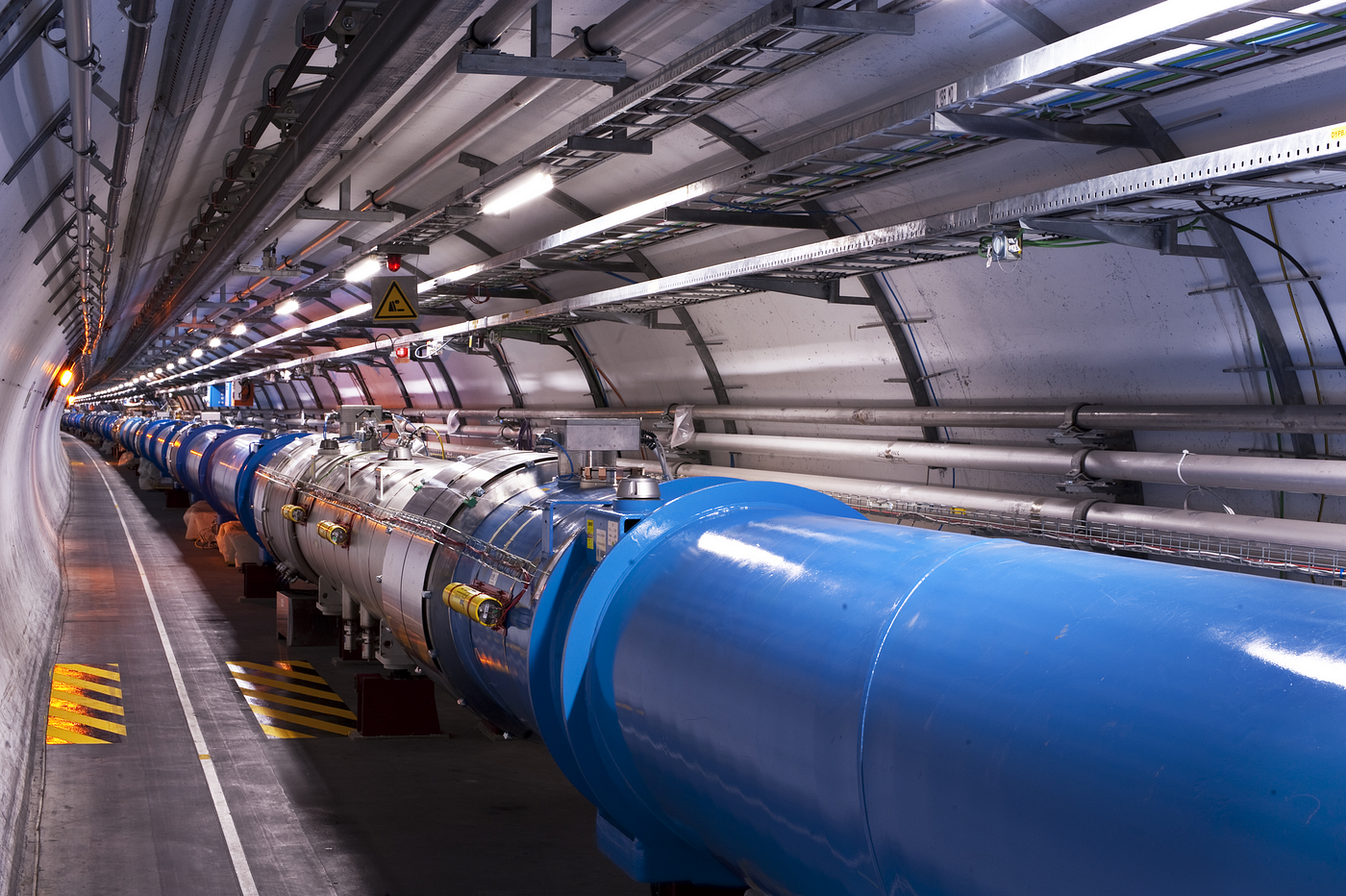An internal view of the ultra-high vacuum tubes that accelerate the particle within the ring | Image credit: CERN

Inside the accelerator, two high-energy proton beams travelling in opposite directions at close to the speed of light are made to collide. These beams of particles smash together and some of the collision energy is converted into mass, creating new particles which are observed in the particle detectors surrounding the collision site.

The particle detector is used to see a wide range of particles and phenomena produced in particle collisions in the LHC. It contains different layers of detectors organized like a cylindrical onion, each of which measures different particles and properties to unprecedented precision. The key data gathered by the particle detector is then analyzed by scientists around the globe to understand what happened at the heart of the collision.

These kinds of collisions and the ensuing data enable us to make tangible progress toward questions like “what is the universe really made of and what are the forces that govern its’ nature?” It’s like getting the opportunity to observe the dawn of the universe — near the Big Bang!

Tackling such fundamental questions are incredibly attractive to grow our basic understanding of the world we live in, and perhaps spark some useful technologies along the way.

For a more detailed explanation of what’s going on at LHC, check out this site to learn more.

## Particle track reconstruction problem description

With a grasp of what’s going on at CERN, let’s define the specific task we’re trying to tackle with quantum graph neural networks.

It turns out that each collision event spawns tens of thousands of particles flying outwards. When these particles pass through the layered detectors, they create signals in the detector called hits. We can expect around 80,000 detector hits from each collision event.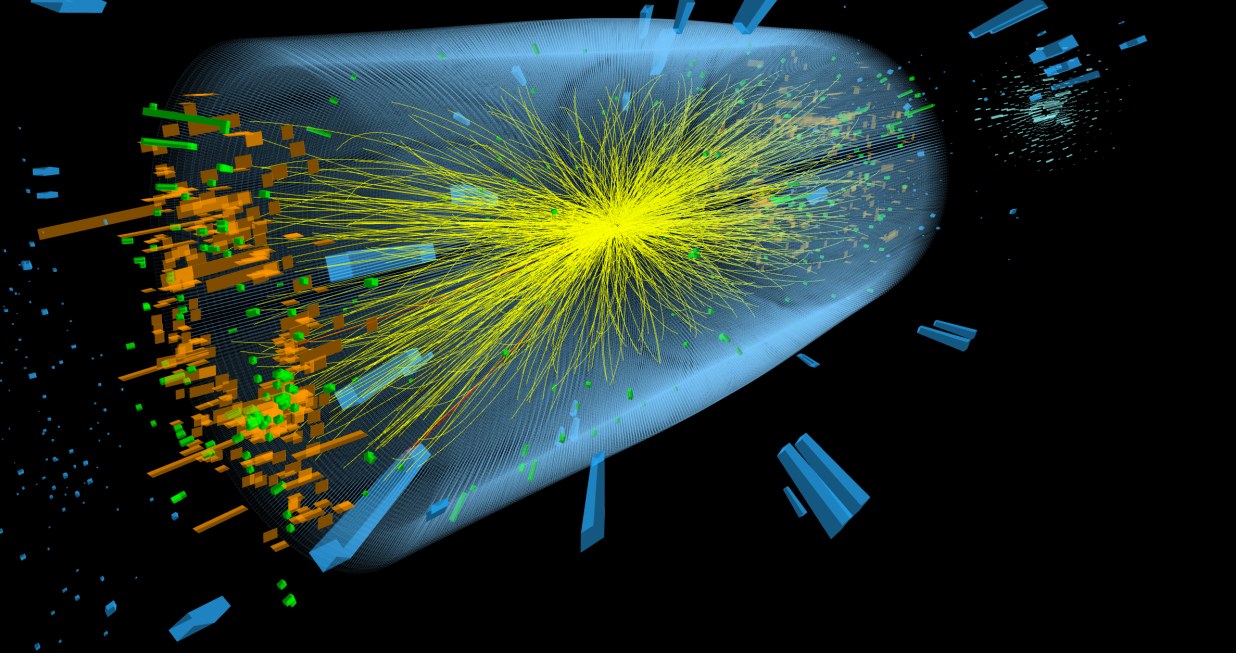A visualization of complex sprays of subatomic particles, produced from colliding proton beams in CERN’s CMS detector at the Large Hadron Collider near Geneva, Switzerland in mid-April of 2018. Credit: CERN

In the particle reconstruction problem, the aim is to connect hits belonging to the same particles so as to accurately identify the true trajectories the individual particles took. When dealing with ~80,000 cumulative hits across all detector layers, however, retracing the paths of each particle grows into an intensive problem.

## Status quo & Opportunity

Right now, computational algorithms for particle reconstruction manage at the current rate of collisions but they suffer from higher collision rates as they scale worse than quadratically (ex. O(n⁶) ). However, CERN is currently upgrading the LHC to have a higher number of particles in each beam (high luminosity) meaning that each collision will spawn even more particles and set off more hits. Scientists realize that the current algorithms will become unwieldy to utilize with the upcoming High Luminosity LHC experiments. So, the efficient reconstruction of particle trajectories is one of the most important challenges in the HL-LHC upgrade.

Given the headwinds current approaches face with scaling, the allure of quantum computing (QC) and its’ recent developments have prompted high energy physicists to explore these computational problems with a new perspective. A lot of effort has been put into inspecting whether new QC tools could be leveraged to gain high speed-ups.

So my team’s QHack project — based upon this paper we read — is focused on applying graph neural networks fused with variational quantum circuits to this problem of particle reconstruction. Let’s dive deeper!

## Graph problem formulation and motivation for graph neural networks

A simple reframing makes it clear how this problem lives squarely within graph theory land.

Think of each detector hit as a node. Then each connection between adjacent layers hits as candidate edges. From here the particle reconstruction problem can be reformulated from a graph perspective as follows.

Given as input the graph of hit nodes, can we devise a neural network to accurately predict the edges of the graph that are true track segments?

If you recall, this is a euphemism for the graph link prediction problem! In essence, the problem is: is there a link between these two nodes? Thus, it is well motivated to employ an edge-level graph neural network.

# Section 2: Classical graph neural networks

We’re now going to take one step back to take two steps forward. As exciting as the problem I just described is, let’s first understand the premise of graph neural networks before biting into the intricacies of the hybrid quantum-classical graph neural network.

If you feel you’re already well-versed in graphML though, feel free to skip this section.

## Graph representation problems

Graphs are everywhere! Graphs are a mathematical object that represents the relations (edges) between a collection of entities (nodes). In other words, it’s a collection of nodes connected by edges.

Each edge or node can be a feature vector of arbitrary dimensions. A wide range of real-world objects/events is often naturally defined in terms of their connections to other objects/events, hence forming a graph.

Examples of graphs in the wild

• Understanding natural language (semantic analysis)
• Traffic data
• Modelling molecules

Though what kind of problems revolve around graphs? Imagine these tasks:

• Given the traffic data across American cities, what is the predicted traffic in New York City tomorrow (node classification)?
• Knowing the network of LinkedIn connections, how likely is it that Henry has already met Terry (link prediction)?
• Given a molecule, what is its’ probability of being toxic (global graph level prediction)?

It doesn’t take much work to see that each of these tasks has an underlying function dictating the so-called “answers”. Aha! Neural networks have proven themselves to be a formidable tool to approximate functions. So is it not reasonable to wonder whether we could figure out a way to leverage neural networks to learn this function and thus attain accurate predictions?

Indeed, this is the objective of graph neural networks! Put simply, they learn to predict certain properties of given graph-structured data. For the sake of exposition, we say that all graph-based tasks fall under the following 3 buckets (see above tasks for corresponding examples):

1. Node-level — predicting some property for each node in a graph
2. Edge-level — predicting the property or presence of edges in a graph
3. Graph-level — predicting a property for a whole graph

## Challenges with graph data

Yet it’s not so trivial. How does a neural network even take a graph as input? Answering this question turns tricky since neural networks have been traditionally used to operate on fixed-size and/or regular-ordered inputs (such as sentences, images and video). So what does it even mean for a neural network to be fed raw graphs as input? Neural networks don’t naturally speak the language of graphs.

We get that it doesn’t work, but what exactly is the issue with inputting graph-structured data?

Graphs are such flexible mathematical representations. So in many problems, the graphs which you process vary by structure. An example case is where the various graphs we train on contain a different number of nodes and edges — like molecules.

We never had to confront this problem when architecting conventional neural networks since the data structure remains consistent among images, audio, or text.

But say we assume that each graph contains a uniform number of nodes, and define a node feature matrix `N` by assigning each node an index `i` and storing the node feature vector `n_i` in `N`. If we do the same with edges, defining an adjacency list storing all edge feature vectors `e_k` connecting nodes `(n_i, n_j )`, we will have achieved a way to represent the graph which is permutation invariant!

That is, assigning a specific index to each edge & node has enabled us to define a regular structure over each graph, one that doesn’t change depending on the order of nodes or edges.

Permutation invariance is a defining characteristic of graph neural networks. It assures that the neural network treats two identical graphs (with superficially different orderings) in the same manner.

For example, the two graphs above are identical yet ordered differently. Indexing has allowed us to see this.

Clearly, if the neural network’s predictions differed between these two graphs, it has not understood that they are identical! Alas, permutation invariance is essential.

Note that many graph instances don’t contain edge feature vectors.

## Naive graph neural network

In the most fundamental sense, a graph neural network learns the properties of graph-structured data. Since indexing can now allow us to package graphs reliably into conventional feed-forward neural networks, we can train a neural network to learn the desired task given proper labels. The simplest of such an architecture could be the following (as detailed here).

Here, unique neural networks are assigned to update each node feature vector, edge feature vector, and an optional global graph node feature vector. After many such simple GNN layers, we arrive at node/edge/graph embeddings which can then be funnelled into a classifier of our choice.

Important aside on embeddings: for the unfamiliar reader, an embedding is a vector with the same size as the feature vector but instead of containing intuitive features that we’ve encoded (ie atom number, traffic volume, etc.), it contains values that densely encode a lot of rich information, despite appearing as gibberish to us. Through several iterations, the embedding vectors evolve to distill core properties of a certain node/edge, enabling a simpler linear classification.

So why is this naive? Well, for one, it’s utterly oblivious to the inherent graph structure of the data. This GNN lacks the concept that neighboring nodes sharing an edge could store critical relationship data in the edge’s embedding. It just doesn’t understand that they’re connected.

One could say that the whole field of machine learning practice is dedicated to exploiting certain regularities within data to better or faster approximate the corresponding function. Along those lines, the neural network above does an abysmal job at incorporating any of such data regularities that could aid in constructing a model architecture. Let’s mention the better approach.

## Graph convolutional neural network (GCN)

I’ve shown you what poorly architected GNNs look like, but what about the good? To arrive there, let’s think through a few questions and reconcile what we already know.

We know that the simple GNN above lacks an understanding of the connected nature of the graph, leading it to learn considerably slower through poor embeddings. So here is the killer question. Is there an embedding procedure particularly well-suited for graph data? Indeed, there is! To introduce it, let’s approach it from a perspective you may find familiar.

As you may know, the state-of-the-art in image classification uses convolutional neural networks (CNNs).

But what makes CNNs so special if image classification is not endemic to them? It’s true that theoretically, any feed-forward neural network could have approximated a classification algorithm using an input layer consisting of a flattened array of pixels from the image.

So why do CNNs work? It’s in the name! Applying learnable convolutional filters across each image enables the network to arrive at rich feature maps. “Rich feature maps” are embeddings after all. These feature maps distill the core attributes of a given image before trying to classify it. This is an enormous advantage.

Essentially, it simplifies the classification problem though compressing the dimensionality of the input data to a rich and digestible size such that it makes it far easier to determine the properties of a certain image.

As you’ll see, the graph neural networks we’ll be dealing with are largely similar. They apply learnable convolutions across each graph to arrive at rich embeddings that are passed through a classification network to determine the properties of a certain graph with relative ease.

These approaches behind convolutions share startling similarities to our new formulation of graph neural networks.

Notice how images are grid-like graphs with regular structure. The pixels are nodes (grayscale floats or RGB vectors) and shared links between each neighbouring pixel form edges (8 each for non-border pixels). Each node also has an absolute position determined by its’ pixel’s x & y coordinate.

So if images are graphs, why can’t we just apply this convolutional technique to our graph neural network?

The topology of our graphs will most likely be devoid of any such uniform rectangular structure, meaning that the shape of a suitable convolutional filter would have to change as it operates across our graphs. For example, one node could have 100 neighboring nodes while the next could have just 1 — what does convolution look like in that case?

It breaks translational invariance, a cornerstone of CNNs, which enforces the same filter is applied across the whole image graph (a cat at the top will be equally identifiable as a cat at the bottom).

Aside from the irregular graph topology, there are also other key differences in the data. Images tend to have much more localized information in comparison to graphs. This matters since a merely 1-hop convolution over an image may provide a meaningful embedding. However, with graphs like social networks, we might require the convolution operations to aggregate information from distant areas of the graph to arrive at meaningful embeddings.

Let’s circle back to our initial question:

Is there a logical embedding procedure that could exploit unique properties of graph-structured data?

Since the above notion of convolutions worked so well for a well-defined structure like an image graph, it’s natural to wonder whether we could generalize convolutions for any graph. This seminal paper presents a core method answering exactly this question. They use the term “message passing” as a supplement to convolutions to graph neural networks.

Message passing

But what is message passing? Modern graph neural networks have adopted a 1-hop localized convolution, and they generally call variations of this approach “message passing”. Similar to a 3x3 image filter in a CNN convolving over 1-hop neighbors: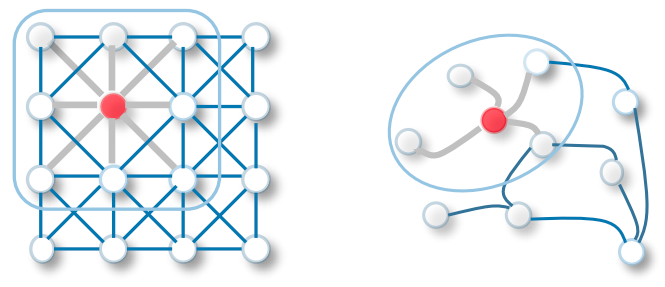Illustration of 2D Convolutional Neural Networks (left) and Graph Convolutional Networks (right), via source

The idea is really simple, each layer of convolutions aggregates information over immediate neighbour nodes `h_u`, for `u ∈ N(h_i)`, with `N(h_i)` being the index set of all neighbouring feature vectors.

It turns out that there’s a key factor that constitutes a convolution in CNNs. It’s the fact that each convolutional filter shares its’ weights when applied across the same image. This makes sense since having it otherwise would imply that one filter would be decoding a plurality of features when applied across the graph. This notion, called weight sharing, is also the case with GCNs. But let’s loop back to understand why this is true later.

GCNs can often be seen as the generalized version of CNNs that can operate on data with underlying non-regular graph structures. So CNNs can be seen as GCNs operating on rectangular graph structures (images).

Graph convolutions

With a sense of how important it is to understand convolutions, let’s dive deeper into them.

The above image demonstrates an individual convolution applied on the top-right node. One round/layer of message passing is when the following is repeated over each node (follow along with the image):

1. Step 1 is a simple calculation where each node aggregates information from surrounding nodes to update its’ embeddings. The feature vectors from each neighboring node of the prior layer are aggregated with the top-right node itself. This is usually through an element-wise sum, mean, or max of all 5 feature vectors. The following is the mathematical representation if we chose to aggregate through calculating the mean: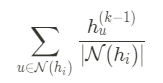Where N(h_i) is the index set of all neighbouring feature vectors to node h_i, and h_u^{(k-1)} is the neighboring node feature vector of the prior layer. It’s also worth noting that |N(h_i)| is the number of neighboring nodes to h_i.

2. Step 2 applies a non-linear (usually learnable) transformation on the aggregated node vector (of step 1) to arrive at the updated embedding. For the purposes of exposition, we choose this transformation to be a trainable weight matrix followed by a ReLU activation (equivalent to a single fully connected neural network layer followed by ReLU).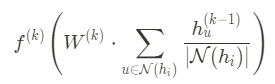Where f^{(k)} and W^{(k)} are the ReLU function and weight matrix of the kth layer, respectively.

The weight matrix is a learnable linear transformation that is applied to each node vector of the layer, and the ReLU activation guarantees some non-linearity. Note that the update function & weight matrix you apply is uniform across all nodes of that layer meaning that the mechanism of embedding is the same which makes sense since even with CNNs the image filter weights are shared across the same layer. To extend this beyond a single-layer-NN, you can use a deeper neural network to construct this non-linear transformation.

3. The subsequent layer’s corresponding node is updated to the resulting feature embedding.

You could also just omit the second step and rely solely on message passing with an aggregation function, but this shrinks the graph neural network to an unlearnable series of aggregations which vastly diminishes its’ power to arrive at meaningful embeddings.

This is basically how a graph convolutional neural network works. Given a graph as input, each graph convolutional layer generates new embeddings for the node & edge vectors — convolving over edge vectors can be easily extended from above despite focusing on nodes — to finally arrive at the final graph embedding. This final embedding can then be fed into any differentiable model (ex. neural network) to make the desired prediction.

Pooling

It’s worth noting that if your graph neural network requires learning edge features, then you will need to pool information from surrounding neighbor nodes into the edge feature vectors using either sum/mean/max.

Pooling can be done before or after the aggregation function for the nodes, but the bottom line is that it must be done to ensure that your edge feature vectors gain awareness of the state of their surrounding nodes.

Pooling procedures are generalized methods to propagate information between edges, nodes, or even global nodes. Here are some examples where each may come of use:

• (Edge→Node) If the task of the GNN is node classification but you have key information stored in edges, then you will need to pool information from the edges into nodes. This is done by aggregating (sum/max/mean) all touching edge feature vectors of a given node and either directly updating the node embedding with the result, or updating the node with the output of an update function that has been fed the aggregated vector.

Conversely, if you only have node features and need to do an edge-level prediction task:

• (Node→Edge) this is the procedure explained at the start of this section.

CNN vs. GCN perspective

To put GCNs into perspective, let’s consider the main differences of a layer’s forward pass:

# Section 3: The hybrid quantum-classical graph neural network (QGNN)

Remember the particle reconstruction problem? Let’s piece through the inner workings of this hybrid quantum-classical graph neural network designed to tackle exactly that.

Recall that the point of our QGNN is to predict the probability that each edge in an inputted event graph is a track segment. Each “event”, being the collision of two particle beams, generates a hit graph of 80,000+ nodes. So at the end of all this, we hope to feed new event graphs into the trained QGNN to infer edge predictions which when connected together form particle trajectories.

The QGNN architecture consists of 3 parts. Follow along using the figure below.

First, cylindrical coordinate information of each hit is sent to an input network that casts it to a higher dimension for embedding. These new embedding vectors are then concatenated (⊕) back with their corresponding cylindrical coordinates to form the initial node feature vectors. We can store each of these initial node vectors in a matrix

where `N_V` is the number of nodes/hits and `N_D` is the number of hidden dimensions that constitute the size of the embedding space that each coordinate was cast to. Note how the matrix is simply an array with each row filled by a nodes’ concatenated feature vector.

Second, the node features are recurrently fed into the same edge networks and node networks, each convolutionally updating the node & edge features (the edge features are simply floats/weights `[0,1]` that describe the probability that the edge under inspection is a track segment). The number of iterations (`N_I`) between the edge and node networks is a hyperparameter that partly dictates the richness of each node embedding. We’ll touch on this afterwards.

Finally, the same edge network is used one last time to obtain the final predicted edge probabilities (`e ∈ [0,1]^{N_E}`), where `N_E` is the number of edges.

Let’s now look deeper into each part of this pipeline.

Note that the edge and node network share weights across layers and within the same layer. This is akin to a convolutional neural network where each convolutional layer uses the same filter as it’s applied across each node of the image. However, what’s different here is that the edge & node networks also share weights across layers since they are recurrently applied. This warrants emphasis: there is only 1 node network and 1 edge network, that is repeatedly applied, so all weights for each of those networks are shared across each application throughout all nodes and layers.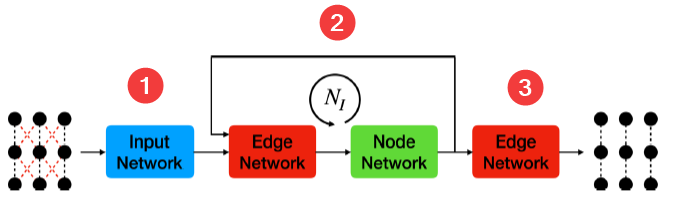The three-step process diagram described above is from : Schematic of the QGNN architecture. The pre-processed graph is fed to an Input Network, which increases the dimension of the node features. Then, the graph’s features are updated with the Edge and Node Networks iteratively, number of iterations (NI ) times. Finally, the same Edge Network is used one more time to extract the edge features of the graph that predicts the track segments. There is only one Edge Network in the pipeline, two Edge Networks are drawn only for visual purposes.

## Input Network

The input network takes as input a matrix of all the hit data per node and applies a single-layer neural network to cast the hit data to an embedding space of a higher dimension. The number of hidden dimensions, `N_D`, is a hyperparameter that dictates the size of all node embeddings.

Where `X` is the input feature matrix, `W^{(i)}` is the weight matrix for the fully connected layer, and `σ` is the activation function.

Input shape — the feature matrix has `N_V` rows and 3 columns (`N_V` being the number of hits/nodes) carrying each hit node vector `[r_i, φ_i, z_i ]` (location in cylindrical coordinates).

Output shape — the output is a matrix of `N_V` rows and `N_D` columns, representing the node embeddings for each hit.

## Edge Network

The edge network is used to predict the probability that a given particle travels from its inner node to the outer node. In other words, it answers the question: does this edge exist? It’s worth noting that these are undirected edges since particles only travel outwards from the origin.

The network takes a pair of node feature vectors as input and returns a float representing the probability that the pair of nodes are connected. Each node feature vector is the concatenation of the 3 fixed spatial coordinates and `N_D` trainable embedding values.

If we let `h_i^(k)` and `h_o^(k)` be the edge’s input and output feature vector at the `k`th layer, respectively, and `e_k` be the returned probability at the `k`th layer, then we can represent the function as:

So let’s zoom into the `EdgeNetwork` function with the help of this diagram taken from the paper.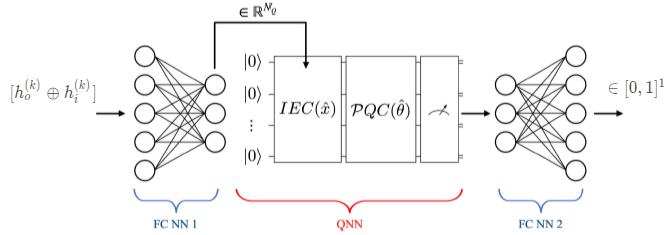The last layer width should be just 1 neuron for the edge network. Adapted from ref paper

Evidently, the edge network is a hybrid quantum-classical neural network. After taking a concatenated vector containing a pair of nodes, the data is fed through a quantum neural network sandwiched in between two trainable classical layers.

Let’s go through this piece by piece. The input classical layer is used to best condense the dimension from `2 × (3+N_D)` to `N_Q`, where `N_Q` is the number of qubits. The classical layer’s `N_Q` outputs are rescaled `[0,π]` which then parameterizes the information encoding circuit (IEC).

Here, the IEC portion of the circuit refers to the information encoding portion which embeds the prior layer’s output vector of dimension `N_Q` (number of qubits) into the quantum neural network. This encoding scheme isn’t trainable, and for our purposes we’ll be using angle embedding meaning we must constrain the prior layer’s outputs to within a π range, accounting for the periodicity of parametric quantum gates. Here we use the RY angle embedding scheme, so the IEC portion looks like this:

Next, the quantum state is evolved under the trainable parameterized quantum circuit (PQC). Then all qubits are measured from the PQC to then be fed into a classical fully connected layer which outputs a single edge probability value using a sigmoid activation. We’ll touch on the circuit structure of the PQC in a bit, but for now, let’s tackle some other things on your mind.

How is the edge network able to learn whether an edge exists given just a pair of nodes?

Each of the node feature vectors contains an embedding that is trained to distill the most helpful information towards making this decision. As you might recall from the graph convolutional neural network, each GNN layer (in our case the node network) is trained to create the best embedding such that the two node embeddings could be used together to decipher whether an edge exists between them or not. We will touch on this in more detail later.

Why not just use a quantum neural network directly?

Sandwiching the quantum neural network in between two classical layers affords us more flexibility with the input and output shape of the aggregate network. Since the input layer size depends heavily on the hidden dimension size, we could easily imagine a scenario where the number of qubits exceeds our ability to simulate comfortably (~20+) if we had used just a quantum neural network.

So having an input classical layer enables us to freely play around with the hidden dimension size while also retaining independent freedom over the number of qubits. Both are interesting hyperparameters that the paper empirically explores.

## Node Network

Since the edge network is the sub-component of the QGNN responsible for predicting track segments, it’s easy to assume that the node network is rather unimportant. This couldn’t be further from the truth. The node network plays a vital role in iteratively updating the node embeddings which condense hidden graph attributes. This allows the edge network to work off of richer hints.

Connecting graph convolutions to the node network

If we think back to the graph convolutional neural network, each convolution used the message passing process paired with a learnable update function to arrive at final node embeddings.

The QGNN node network achieves equivalent aims but in a slightly different manner. Instead of feeding 1 aggregated node vector to the update function (trainable neural network) like in the GCN, our node network accepts 3 nodes vectors. 2 are aggregated based upon whether the neighbor is in a prior or subsequent layer, and the third is the target node vector itself.

Input / Output Inductive Bias

To get a clearer picture of what’s going on, let’s dive deeper into the I/O.

The node network takes as input a concatenated triplet of 3 node feature vectors (achieving a fixed value as needed). The first — `h’_{j,input}` — is a weighted sum over all neighboring node features residing in the detector layer before the `h_j` layer. The second — `h’_{j,output}` — is a weighted sum over all neighboring node features that lie in a detector layer directly ahead of the `h_j` layer, and the last being the target node feature vector itself — `h_j`.

To make this concrete, let’s inspect the mathematical definition:

Where `N_{input}(h_j)` is the index set of all neighboring node feature vectors located in 1 detector layer prior to `h_j` and similarly`N_{output}(h_j)` is the index set of all neighboring nodes located 1 detector ahead of `h_j`. `e_{ij}` is the edge weight assigned by prior edge network execution on the edge connecting `h_i` and `h_j`.

It’s worth noting that incorporating knowledge about our specific problem’s graph structure (hits in the form of detector layers) into the node network architecture is an inductive bias that helps us make a more suitable neural network.

Attention passing

Q: Why is the sum weighted? And why are the weights edge probabilities?

Weighted sums allow us to vary the influence each neighboring node has on the target node in question.

Specifically, we notice that the weights are the edge probabilities corresponding to the edge between `h_i `and `h_j`. This is more profound than what meets the eye. We’re effectively using the edge network’s predictions within the QGNN itself to hint to the node network as to which neighboring nodes to pay more or less attention to.

The likelier a neighboring node is to form a track segment with `h_j`, the stronger influence that neighboring node has on `h_j`’s final embedding.

So effectively, the edge network serves as an attention model that feeds information to the node network on which surrounding nodes are important to attend to (hence the name attention model)! This in turn helps the edge network identify the existence of a track segment since there’s a higher correlation between two nodes that are likelier to form an edge.

Architecture

Having understood the attention mechanism and the input components, let’s knit these together and understand what lies inside the node network function below.

As can be seen, the hybrid quantum-classical neural network architecture is almost the same as the edge network except for the input and output classical layers. The input dimension is `3 × (3+N_D)` and the output is the node embedding of dimension `N_D`.

Apart from that, the encoding scheme (IEC) remains a RY angle embedding, and the parameterized quantum circuit is trainable with weights `θ` designated for the node network.

The encapsulating classical fully connected layers are also trainable and are there for the same reason as they were in the edge network — to enable full flexibility in the hidden dimension size and the number of qubits.

For more detail on the data flow through the network, refer back to the explanation given in the edge network section. Let’s now focus instead on the quantum computing aspect of the hybrid quantum-classical neural network.

## Ansatz choice

Heretofore, we briefly addressed the PQC but never saw what the circuit actually looks like. Partly since it can largely vary!

Most obviously, the width of the circuit varies per the hyperparameter `N_Q`. But less trivial is that there still remains a lot of active research being done to understand which ansatze (parameterized quantum circuit structures) perform best within quantum neural networks.

For the purposes of exposition, we’ll avoid this can of worms for now and instead I’ll show a few examples of ansatze that work generally well.

The first one below is from Sim2019, while the other was made by one of my team members at QHack! Interestingly, we found that the latter was more robust to Barren Plateaus when training the QGNN (measured using the gradient variance across each layer)!

One metric we used to evaluate the strength of an ansatz is its’ proneness to vanishing gradients. We found that a leathered entanglement structure, like that of `circuit10` and the second ansatz below generally tended to produce better results.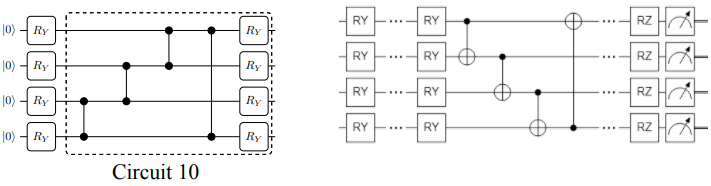[Left] Single layer of circuit 10 from Sim2019 ; [Right] Single layer of the ansatz developed during QHack

## Training and loss function

Once you’ve understood how a QGNN works, training it is rather simple. The process is very similar to any other binary classification model, with the addition that the quantum gradients need to be calculated independently using inefficient analytic parameter-shift rules.

To train the QGNN:

1. Feed-in a batch of events (individual graphs of hits), and conduct a forward pass to arrive at the predicted edge structure for each event.
2. Calculate the binary cross-entropy loss between the predicted edge values and the labels. See below for the mathematical formulation.
3. Backpropagate the loss to find the gradients of each classical and quantum weight. The quantum gradients are analytically found using parameter-shift rule. The classical gradients are computed as usual leveraging auto-differentiation.
4. Update each weight using calculated gradients with the optimizer of your choice.  uses an ADAM optimizer with a learning rate of 0.01.
5. Repeat above for all batches in an epoch, over 10–100 epochs.

(2 extended) Like several other binary classification models, we train the QGNN using the binary cross-entropy loss function, where `y_i` is the truth label and `hat{y_i}` is the model prediction for edge `i`.

## Recapping QGNNs

Equipped with a deeper understanding of the components, let’s recap how they all mesh together and touch on the motivation behind some design choices.

Here’s a high-level distillation of how the QGNN conducts one forward pass and its’ significance.

Once each hit’s spatial coordinates have been inputted, the InputNetwork casts them to an embedding space of `N_D` dimensionality. Then, the node embeddings concatenated with their respective spatial coordinates are recurrently fed through the edge network, then the node network, repeatedly for `N_I` iterations. The node’s latent variables (embeddings in `N_D`) and edge weights are alternatively updated with each pass-through of the constituent networks.

The need for iterations

Each new iteration brings a new cycle of convolutions. With greater iterations, information is propagated to farther nodes. This enables the node embeddings to capture broader graph properties, allowing it to update a node’s local features with wider awareness.

Concretely, if one pass through the node network embeds information from a 1-hop neighborhood, the second pass of the node network would expand each node’s influence to a 2-hop radius and so on.

The need for recurrence

One may ask why we chose to use a single edge network & node network throughout the QGNN, instead of using multiple. Take a look at what Cenk (lead author) from  said as I mentioned this:

Using different layers would be very expensive. It would require more resources to train and probably more epochs as well due to `N_I` times increase in number of paramaters.
— Cenk Tüysüz (lead author of
)

He means to say that training with respect to each parameter would become unwieldy if we had used different edge & node networks with each iteration through the pipeline.

The edge & node network interplay

We understand that the graph is updated through each node & edge network, but how exactly do they play off of each other? Let’s address that.

The edge network serves as an attention model for the node network. It feeds information to the node network through its’ edge weights on which surrounding nodes are important to pay attention to.

The node network then uses the help of the hints given by the edge network when aggregating neighborhood nodes to best arrive at node embeddings capturing hidden attributes of the graph. The embedding is stored within the hidden dimensions of each node vector. Being the only inputs, these latent embeddings then end up directly contributing to the edge network’s ability to distinguish between the nodes that are connected and those that are not.

After a few iterations of that, the edge network is used one last time on the best node embeddings to arrive at the final edge predictions on the inputted event graph.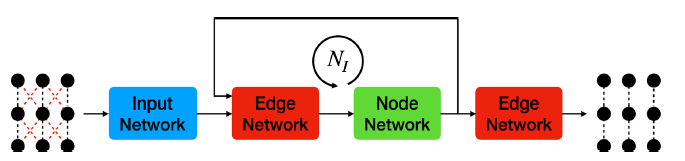There is only one Edge Network in the pipeline, two Edge Networks are drawn only for visual purposes.

To crystallize the process, let’s compare 3 essential aspects between the QGNN and the GCNN we covered earlier:

# Section 4: Architecture improvements made in QHack

Thus far, I’ve detailed at great length how the original QGNN works as brought forth within .

In this optional section, I’ll detail how my team and I extended the work to expand the ability of this specific QGNN.

A known issue of recurrent neural networks is the vanishing gradient problem and the QGNN used here is no exception. Training an RNN requires using back-propagation through time (BPTT), which means that you choose a number of time steps `N`, and you unroll your network such that it becomes a feed-forward neural network (FFNN) with `N` duplicates of the original network.

Changing the activation function improved the vanishing gradient problem

Parsing through the existing QGNN architecture, our team noticed the prevalence of sigmoid activation functions throughout all layers of the network.

Naturally, we wondered why. After speaking with the lead author of the original paper, we realized that it was simply used to meet the parameter constraints of quantum gates, `[-π, π]`.

Further, due to the `2π` periodicity of these quantum gates, the quantum parameters must be constrained within `[0, π]`. Such a constraint is effectively achieved by applying a `π` factor to the output of each sigmoid. So we understood that the motivation behind the use of sigmoid activation functions throughout the network was to adhere to the `[0,1]` bounds.

However, it’s well understood in classical machine learning literature that sigmoid activations in between layers can often lead to vanishing gradients, and this is only exacerbated by the recurrence!

As you can see, the gradient for the sigmoid function will saturate and when using the chain rule, it will shrink. By contrast, the derivative for the rectified linear unit (ReLU) is always 1 or 0. The argument for using ReLU activation functions within the hidden layers is made even stronger when considering that ReLU remains the most widely used activation function in classical graph neural networks.

So, motivated by the appeal of ReLU, our group probed the question of whether it is possible to adapt a ReLU function to the QGNN such that one can both benefit from the rich gradients that ReLU provides while conforming to the `[0,1]` output bounds that are required for all classical layers that feed into a parameterized quantum circuit.

In this project, we proved the affirmative. We substituted all of the sigmoid activation functions except for the edge network outputs with ReLU activations that are paired with a rescaling layer which is used to re-scale all tensor values to be in `[0,1]`.

By employing this technique, the QGNN was immune to vanishing gradients for up to 50 more layers in comparison to the original architecture based upon the sigmoid activation.

I’m also excited to share that these changes have now been merged into the main project repository!

## Assumptions, gaps & future work

• Assumptions — The 5 day time constraint meant that we could never train the complete QGNN over the complete set of all events (takes a whole week to train). What this implies is that there’s a chance that our findings with the vanishing gradient problem don’t necessarily pan out somewhere along the way when fully training.
• Future work — There still remains a lot of tinkering that can be done with the architecture of this primitive QGNN to see how performance could be improved. Specifically, it would interest me to see the performance on a GNN with a more conventional message passing & aggregation method.

If you enjoyed the article or learned a ton, feel free to reach me at pavanjay.com or @pavanjayasinha.

--

--